## 中文分词算法 之 基于词典的逆向最大匹配算法 原荐

``````    public static List<String> segReverse(String text){
Stack<String> result = new Stack<>();
while(text.length()>0){
int len=MAX_LENGTH;
if(text.length()<len){
len=text.length();
}
//取指定的最大长度的文本去词典里面匹配
String tryWord = text.substring(text.length() - len);
while(!DIC.contains(tryWord)){
//如果长度为一且在词典中未找到匹配，则按长度为一切分
if(tryWord.length()==1){
break;
}
//如果匹配不到，则长度减一继续匹配
tryWord=tryWord.substring(1);
}
result.push(tryWord);
//从待分词文本中去除已经分词的文本
text=text.substring(0, text.length()-tryWord.length());
}
int len=result.size();
List<String> list = new ArrayList<>(len);
for(int i=0;i<len;i++){
list.add(result.pop());
}
return list;
}``````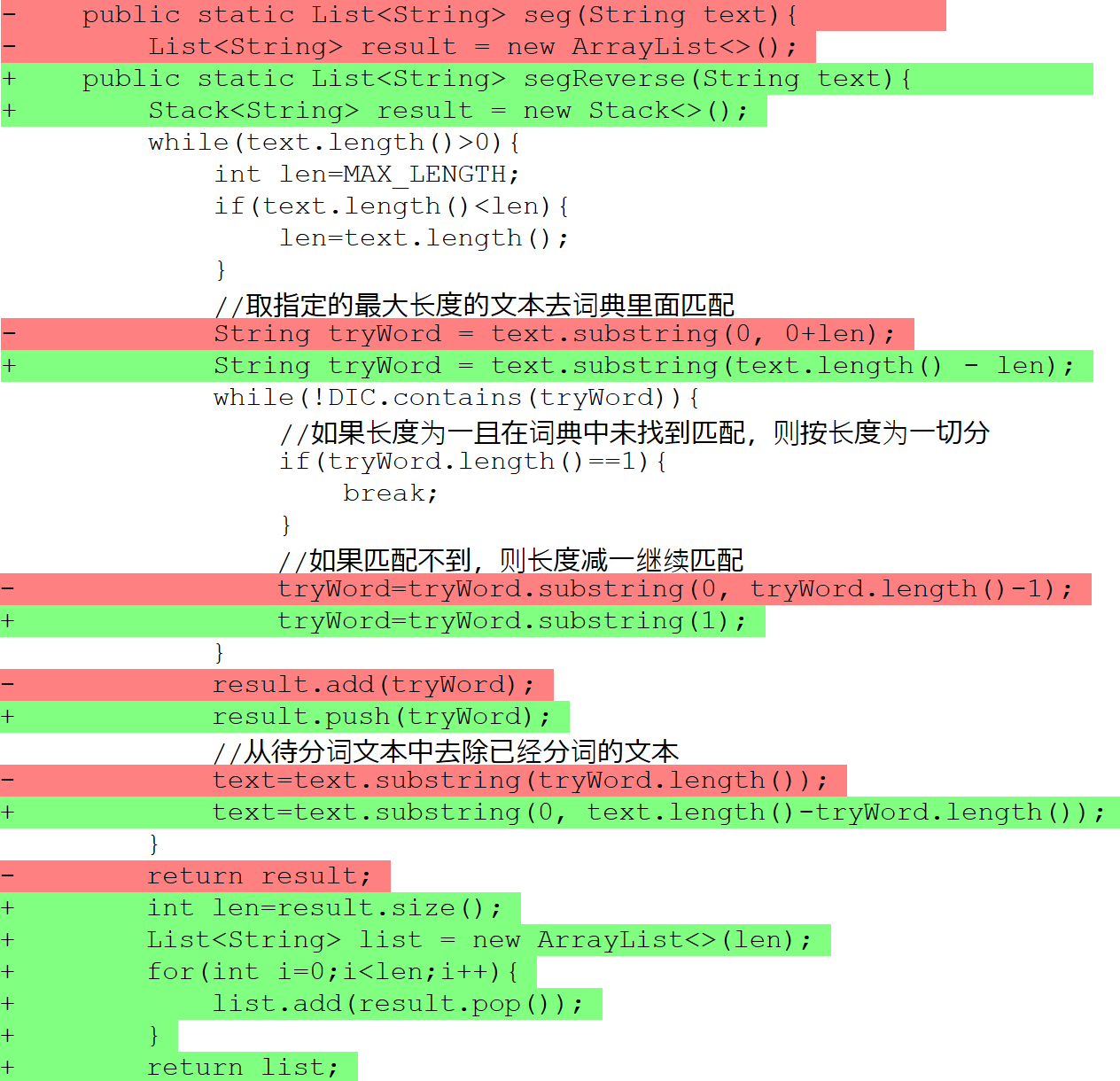``````public static void main(String[] args){
List<String> sentences = new ArrayList<>();
sentences.add("杨尚川是APDPlat应用级产品开发平台的作者");
sentences.add("研究生命的起源");
sentences.add("长春市长春节致辞");
sentences.add("他从马上下来");
sentences.add("乒乓球拍卖完了");
sentences.add("咬死猎人的狗");
sentences.add("大学生活象白纸");
sentences.add("他有各种才能");
sentences.add("有意见分歧");
for(String sentence : sentences){
System.out.println("正向最大匹配: "+seg(sentence));
System.out.println("逆向最大匹配: "+segReverse(sentence));
}
}``````

``````开始初始化词典

``````/**
* 将一个文件分词后保存到另一个文件
* @author 杨尚川
*/
public class SegFile {
public static void main(String[] args) throws Exception{
String input = "input.txt";
String output = "output.txt";
if(args.length == 2){
input = args;
output = args;
}
long start = System.currentTimeMillis();
segFile(input, output);
long cost = System.currentTimeMillis()-start;
System.out.println("cost time:"+cost+" ms");
}
public static void segFile(String input, String output) throws Exception{
float max=(float)Runtime.getRuntime().maxMemory()/1000000;
float total=(float)Runtime.getRuntime().totalMemory()/1000000;
float free=(float)Runtime.getRuntime().freeMemory()/1000000;
String pre="执行之前剩余内存:"+max+"-"+total+"+"+free+"="+(max-total+free);
try(BufferedReader reader = new BufferedReader(new InputStreamReader(new FileInputStream(input),"utf-8"));
BufferedWriter writer = new BufferedWriter(new OutputStreamWriter(new FileOutputStream(output),"utf-8"))){
int textLength=0;
long start = System.currentTimeMillis();
String line = reader.readLine();
while(line != null){
textLength += line.length();
writer.write(WordSeg.seg(line).toString()+"\n");
line = reader.readLine();
}
long cost = System.currentTimeMillis() - start;
float rate = textLength/cost;
System.out.println("文本字符："+textLength);
System.out.println("分词耗时："+cost+" 毫秒");
System.out.println("分词速度："+rate+" 字符/毫秒");
}
max=(float)Runtime.getRuntime().maxMemory()/1000000;
total=(float)Runtime.getRuntime().totalMemory()/1000000;
free=(float)Runtime.getRuntime().freeMemory()/1000000;
String post="执行之后剩余内存:"+max+"-"+total+"+"+free+"="+(max-total+free);
System.out.println(pre);
System.out.println(post);
}
}``````

``````开始初始化词典
dic.class=org.apdplat.word.dictionary.impl.TrieV3
dic.path=dic.txt

cost time:64029 ms``````

``````开始初始化词典
dic.class=org.apdplat.word.dictionary.impl.HashSet
dic.path=dic.txt

cost time:77271 ms``````

``````public static List<String> seg(String text){
List<String> result = new ArrayList<>();
while(text.length()>0){
int len=MAX_LENGTH;
if(text.length()<len){
len=text.length();
}
//取指定的最大长度的文本去词典里面匹配
String tryWord = text.substring(0, 0+len);
while(!DIC.contains(tryWord)){
//如果长度为一且在词典中未找到匹配，则按长度为一切分
if(tryWord.length()==1){
break;
}
//如果匹配不到，则长度减一继续匹配
tryWord=tryWord.substring(0, tryWord.length()-1);
}
result.add(tryWord);
//从待分词文本中去除已经分词的文本
text=text.substring(tryWord.length());
}
return result;
}``````

``````public static List<String> seg(String text){
List<String> result = new ArrayList<>();
//文本长度
final int textLen=text.length();
//从未分词的文本中截取的长度
int len=DIC.getMaxLength();
//剩下未分词的文本的索引
int start=0;
//只要有词未切分完就一直继续
while(start<textLen){
if(len>textLen-start){
//如果未分词的文本的长度小于截取的长度
//则缩短截取的长度
len=textLen-start;
}
//用长为len的字符串查词典
while(!DIC.contains(text, start, len)){
//如果长度为一且在词典中未找到匹配
//则按长度为一切分
if(len==1){
break;
}
//如果查不到，则长度减一后继续
len--;
}
result.add(text.substring(start, start+len));
//从待分词文本中向后移动索引，滑过已经分词的文本
start+=len;
//每一次成功切词后都要重置截取长度
len=DIC.getMaxLength();
}
return result;
}
public static List<String> segReverse(String text){
Stack<String> result = new Stack<>();
//文本长度
final int textLen=text.length();
//从未分词的文本中截取的长度
int len=DIC.getMaxLength();
//剩下未分词的文本的索引
int start=textLen-len;
//处理文本长度小于最大词长的情况
if(start<0){
start=0;
}
if(len>textLen-start){
//如果未分词的文本的长度小于截取的长度
//则缩短截取的长度
len=textLen-start;
}
//只要有词未切分完就一直继续
while(start>=0 && len>0){
//用长为len的字符串查词典
while(!DIC.contains(text, start, len)){
//如果长度为一且在词典中未找到匹配
//则按长度为一切分
if(len==1){
break;
}
//如果查不到，则长度减一
//索引向后移动一个字，然后继续
len--;
start++;
}
result.push(text.substring(start, start+len));
//每一次成功切词后都要重置截取长度
len=DIC.getMaxLength();
if(len>start){
//如果未分词的文本的长度小于截取的长度
//则缩短截取的长度
len=start;
}
//每一次成功切词后都要重置开始索引位置
//从待分词文本中向前移动最大词长个索引
//将未分词的文本纳入下次分词的范围
start-=len;
}
len=result.size();
List<String> list = new ArrayList<>(len);
for(int i=0;i<len;i++){
list.add(result.pop());
}
return list;
}``````

``````开始初始化词典
dic.class=org.apdplat.word.dictionary.impl.TrieV3
dic.path=dic.txt

cost time:25007 ms``````

``````开始初始化词典
dic.class=org.apdplat.word.dictionary.impl.HashSet
dic.path=dic.txt

cost time:40928 ms``````

``````public class HashSet implements Dictionary{
private Set<String> set = new java.util.HashSet<>();
private int maxLength;
@Override
public int getMaxLength() {
return maxLength;
}
@Override
public boolean contains(String item, int start, int length) {
return set.contains(item.substring(start, start+length));
}
@Override
public boolean contains(String item) {
return set.contains(item);
}
@Override
public void addAll(List<String> items) {
for(String item : items){
add(item);
}
}
@Override
public void add(String item) {
//去掉首尾空白字符
item=item.trim();
int len = item.length();
if(len < 1){
//长度小于1则忽略
return;
}
if(len>maxLength){
maxLength=len;
}
set.add(item);
}
}``````

JDK的HashSet没有这里优化所使用的contains(String item, int start, int length)方法，所以用了substring，这是HashSet提速没有TrieV3大的原因之一。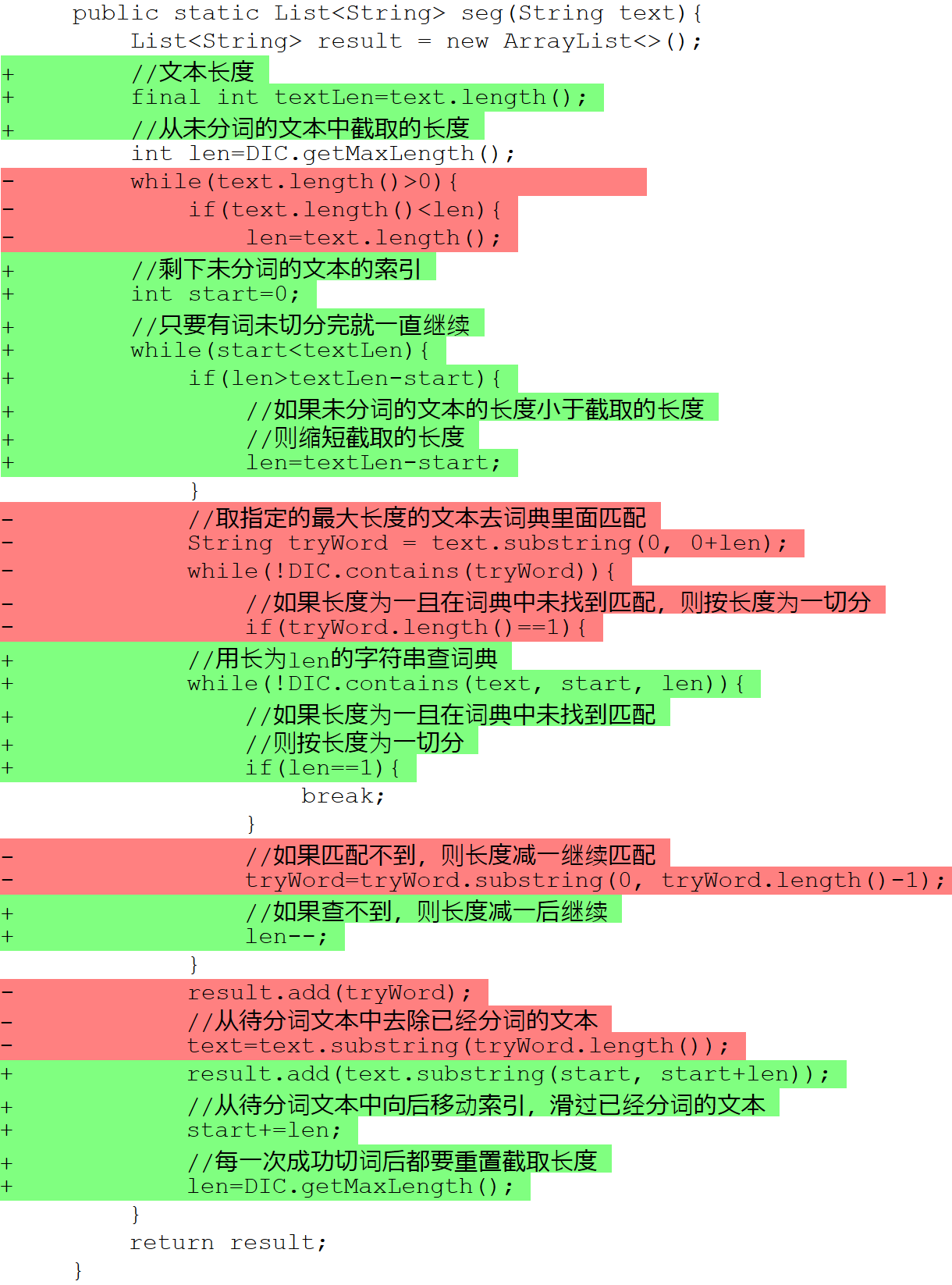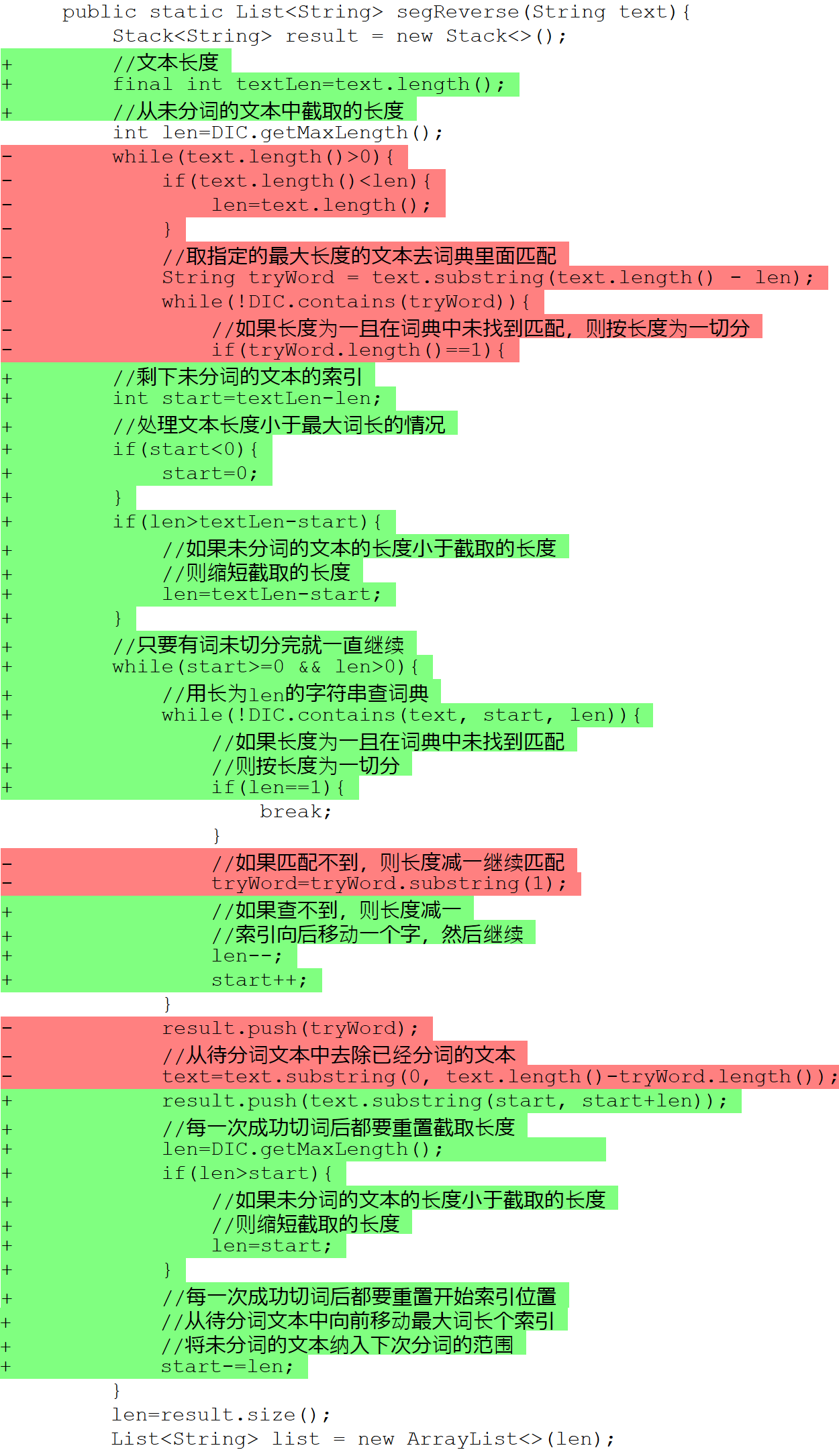1、中文分词十年回顾

NUTCH/HADOOP视频教程

© 著作权归作者所有### 杨尚川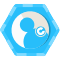### 评论(1)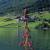2014/04/04
916
0
Java中文分词组件 - word分词

Java分布式中文分词组件 - word分词 word分词是一个Java实现的分布式的中文分词组件，提供了多种基于词典的分词算法，并利用ngram模型来消除歧义。能准确识别英文、数字，以及日期、时间等数...

2014/04/29
24.9K
56

2014/03/28
1K
0

wangtaotao
2014/04/06
0
0
NLP系列-中文分词（基于词典）

hiyoung
2018/09/20
0
0

Spring boot 静态资源访问

0. 两个配置 spring.mvc.static-path-patternspring.resources.static-locations 1. application中需要先行的两个配置项 1.1 spring.mvc.static-path-pattern 这个配置项是告诉springboo......

moon888

3
0
hash slot（虚拟桶）

4
0
Kafka 原理和实战

vivo互联网技术

19
0
java数据类型

audience_1

9
0

Java技术剑

27
0